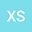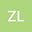Existence solutions of integral equation and initial value questions for differential equation of fractional order
••Xiaoyan Shi
Southwestern University of Finance and Economics

Corresponding Author:[email protected]

Author Profile## Abstract

In this paper, using the method of upper and lower solutions and fixed point Theorem, consider the existence result of the initial value problem for fractional differential equation of Riemann-Liouville fractional derivative, obtained the existence results for maximal and minimal solutions.